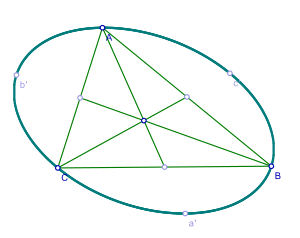# Steiner ellipseThe Steiner ellipse of an isosceles triangle. The three line segments inside the triangle are the triangle's medians, each bisecting a side. The medians coincide at the triangle's centroid, which is also the center of the Steiner ellipse.

In geometry, the Steiner ellipse of a triangle, also called the Steiner circumellipse to distinguish it from the Steiner inellipse, is the unique circumellipse (ellipse that touches the triangle at its vertices) whose center is the triangle's centroid. Named after Jakob Steiner, it is an example of a circumconic. By comparison the circumcircle of a triangle is another circumconic that touches the triangle at its vertices, but is not centered at the triangle's centroid unless the triangle is equilateral.

The area of the Steiner ellipse equals the area of the triangle timesand hence is 4 times the area of the Steiner inellipse. The Steiner ellipse has the least area of any ellipse circumscribed about the triangle.

## Trilinear equation

The equation of the Steiner circumellipse in trilinear coordinates isfor side lengths a, b, c.

## Axes and foci

The semi-major and semi-minor axes have lengthsand focal lengthwhereThe foci are called the Bickart points of the triangle.

## Cartesian coordinates

Given a triangle with vertices,

the linear problem,

can be solved, and the reciprocals of the eigenvalues of the matrix form of the solutionare 3 times the squared lengths of the semi-major axis and semi-minor axis; the corresponding eigenvectors relate to the orientation. This approach generalizes to higher dimensions.

## References

1. Weisstein, Eric W. "Steiner Circumellipse." From MathWorld—A Wolfram Web Resource. http://mathworld.wolfram.com/SteinerCircumellipse.html